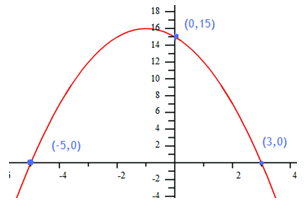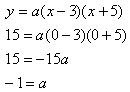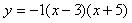Graphs
Example 2

Find the equation to model the graph below:Step 1. Choose the appropriate form.

For this graph, the x-intercepts are known so factored form is appropriate.

Step 2. Substitute the x-intercepts into the formula to find the a-value.Step 3. Write a model.